Levels »

# A Plague of Demons

### The Catacombs, Elevation: -4.5m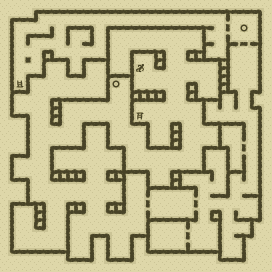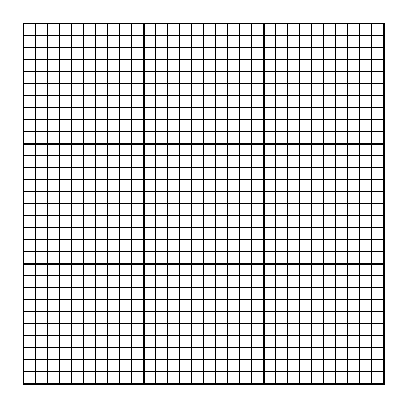### Items

•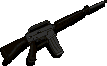Broken M-16 (16, 7) (on John)
•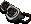Infra-Red Goggles (16, 7) (on John)
•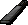2 MP-41 Magazines (64 rounds) (11, 7) x32 - (1, 25) x32
•2 M-16 Magazines (0 rounds) (16, 7) x0 (in Broken M-16) (on John) - (16, 7) x0 (on John)
•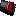40mm HE Cartridge (16, 7) (on John)
•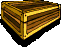Cedar Box (18, 28)

•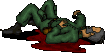(16, 7) John
•Broken M-16
•M-16 Magazine x0
•M-16 Magazine x0
•Infra-Red Goggles
•40mm HE Cartridge

### Monsters

•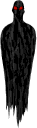3 Phantasms (9, 3) - (28, 3) - (28, 15)
•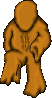2 Oozes (1, 20) - (29, 27)
•55 Invisible Wraiths (Random rate: 7) (6, 1) - (11, 1) - (12, 1) - (28, 1) - (26, 2) - (30, 3) - (6, 4) - (23, 4) - (3, 5) - (17, 6) - (11, 7) - (14, 7) - (5, 8) - (10, 8) - (13, 9) - (18, 9) - (13, 10) - (24, 10) - (1, 12) - (12, 12) - (19, 13) - (23, 13) - (13, 15) - (25, 15) - (4, 16) - (10, 16) - (7, 18) - (16, 18) - (1, 19) - (7, 19) - (13, 19) - (15, 19) - (25, 19) - (18, 21) - (19, 21) - (12, 23) - (20, 23) - (21, 23) - (22, 23) - (24, 23) - (21, 24) - (22, 24) - (1, 25) - (20, 25) - (21, 25) - (22, 25) - (24, 25) - (30, 25) - (2, 26) - (21, 27) - (4, 28) - (9, 29) - (13, 29) - (21, 29) - (28, 30)

### Locations

• 9 Door openers (26, 2) - (28, 2) - (29, 3) - (29, 5) - (30, 17) - (16, 24) - (18, 24) - (22, 24) - (24, 24)
• Silver key-operated door opener (23, 28)
• 2 Save runes(29, 2) - (13, 9)